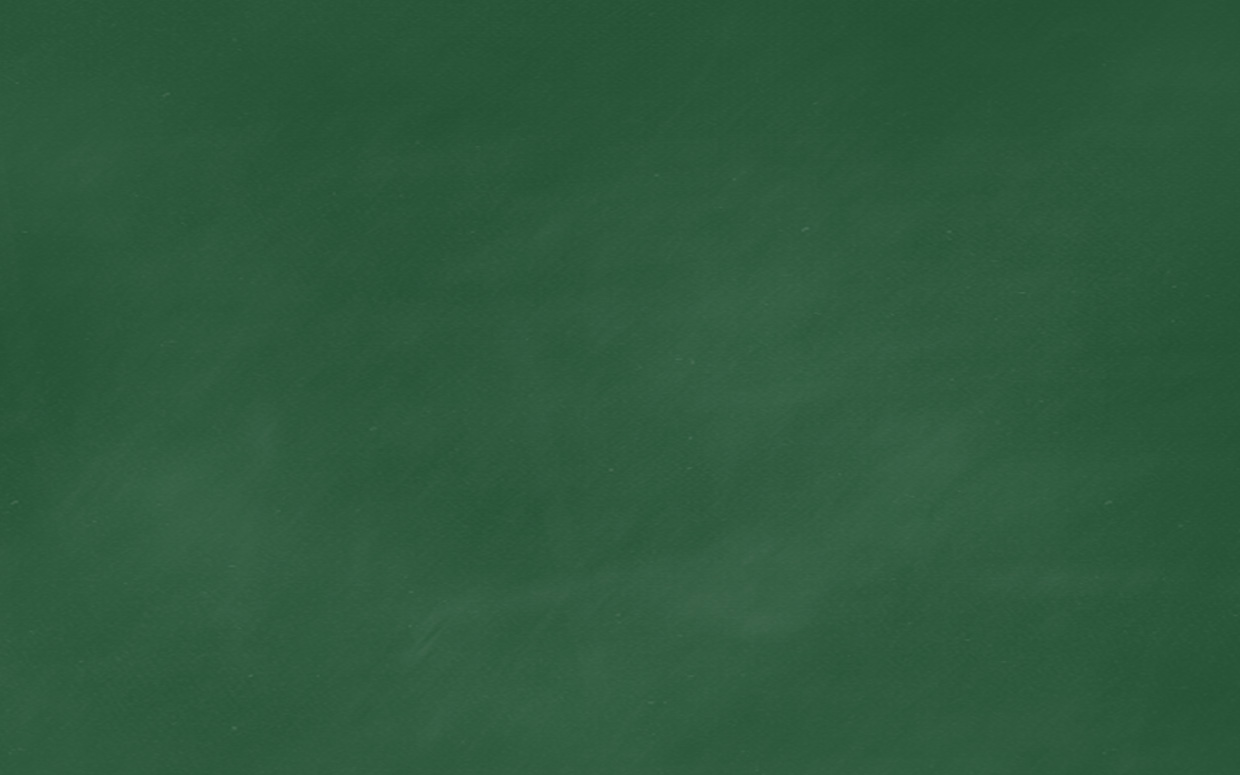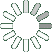Puzzle Command
Daily Puzzle
Number Logic #20
March 15, 2015Find a 6 digit number where:

The 1st digit is greater than 4 and lesser than 9.
The 2nd digit is the number of 1s in the number.
The 3rd digit is the number of 3s in the number.
The 4th digit is the number of 5s in the number.
The 5th digit is equal to 5.
The 6th digit is equal to 1.

All digits are odd.

Puzzle Notes
6 digit numbers range from 100,000 to 999,999.
Enter in your solution below and click submit.